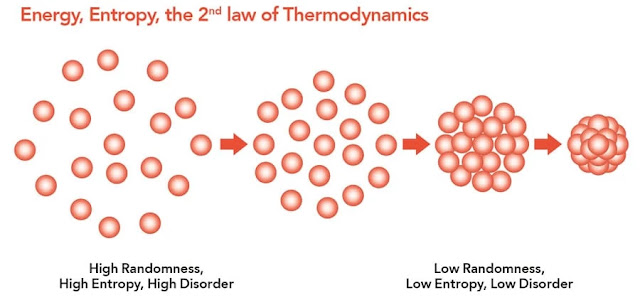### Delta S in Chemistry

Delta S is a measure of entropy within a system. This refers to the amount of random motion occurring in a certain medium. Delta S is mostly famously referred to within the Second Law of Thermodynamics which states that the entropy of the universe is increasing over time (always increases for spontaneous processes). The delta within Delta S is the uppercase Greek letter Δ, the fourth letter within the Greek alphabet.Understanding Delta S (entropy) through a particle collection. Source
The diagram above explains Delta S within a collection of particles. If they are tightly ordered and packed together, then the value of Delta S is lower to reflect this order. On the flip side, if they have space to move around at random (being farther apart) then the value of Delta S is higher because their is a large amount of disorder. The concrete example below should help clarify any misunderstandings.Collage of disordered legos and ordered finished lego build.
There is an obvious juxtaposition between the disorder of the free lego blocks and the order of the finished build in the top right hand corner. The free legos have a high degree of randomness and therefore a higher value for Delta S. The completed lego set is far more ordered and therefore has a lower value for Delta S. Many actions that we take as humans to order our lives are in service of lowering entropy; however, many of notice that Delta S naturally increases as a function of time. We also know that it takes energy in order to turn a disordered mess into an ordered product.

How are energy and entropy (Delta S) related?Gibb's Free Energy Equation. Source
The two concepts are related via the Gibb's free energy equation which is specifically utilized for chemical reactions in order to determine whether they are spontaneous or nonspontaneous. This refers to whether they will happen without requiring an energy input. If a reaction has a high Delta S then it is more likely to be spontaneous. Whether Delta S is positive or negative is often measured based on the number of particles on either side of the chemical equation.

But back to energy - the concept is first related to heat transfer also represented here by Delta H or enthalpy. This is specific to different actions/reactions but is a type of energy transfer. Forming bonds typically has a negative enthalpy whereas breaking bonds is associated with a positive enthalpy. This is because energy is required in order to break a bond and thereby released when a bond is reformed.Thermometer. Source
The second way energy applies is also related to Delta S. Temperature is a measure of the average kinetic energy within the molecules in the surrounding atmosphere. As the temperature increases more energy is available from the surroundings to facilitate a reaction if necessary. As is shown in the equation temperature is multiplied by the value of Delta S.

These two finally relate via the Gibb's Free Energy value. If G is positive, then the reaction will not proceed until an outside source of energy is found. If G is negative, then the reaction will proceed spontaneously and entropy (Delta S) will be increased.